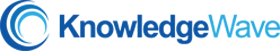## Excel 102: Plan a Worksheet and Learn Basic Formulas

In this live training event, Excel novices will learn how to create a spreadsheet, build basic formulas, and format a worksheet.

Recommended Duration: 45-minutes + Q & A

## Core Objectives

• Learn the steps of the spreadsheet creation process
• Create and save a new sheet
• Create formulas from scratch
• Insert columns and rows
• Change the size of columns and rows
• Create formulas using the mathematical order of operations
• Format text and numbers